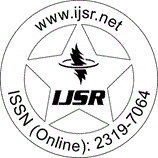International Journal of Science and Research (IJSR)
Call for Papers | Fully Refereed | Open Access | Double Blind Peer Reviewed

Downloads: 2 | Views: 47 | Weekly Hits: ⮙1 | Monthly Hits: ⮙2

Research Paper | Mathematics | Cameroon | Volume 11 Issue 8, August 2022

# Optimal Investment under Partial Information in a Portfolio with Three Financial Assets

Tatiagoum K. Eric

Abstract: In this work, we solve the problem of optimal investment for the hedging of a European option with a portfolio made up of three financial assets: A risky asset of price St, an asset whose price St? is a deterministic function of a stochastic interest rate rt and finally, a non-risky asset of price St0. We assume that the payoff ? of the option at maturity date T does not only depend on the strike price ST of the underlying risky asset but also on an unobservable random variable B. We put ourselves in the situation where the short term interest rate (rt)0?t?T is rather a stochastic variable. The two observable variables are: The price of the risky asset St and the interest rate rt. We propose to determine a vector (u??)0?t?T = (? ut, u?0t)0?t?T of optimal strategies giving at each date t before maturity the optimal amount of assets to invest in the portfolio. We base our determination on maximizing the utility of the terminal wealth of the portfolio. We first transform the problem into a full information problem using the theory of filtering with the stochastic partial differential equations (SPDE).

Keywords: Optimal investment, stochastic maximum principle, three assets, partial information

Edition: Volume 11 Issue 8, August 2022,

Pages: 899 - 916